# RS Aggarwal Class 9 Solutions Chapter 1 Real Numbers Ex 1F

## RS Aggarwal Class 9 Solutions Chapter 1 Real Numbers Ex 1F

These Solutions are part of RS Aggarwal Solutions Class 9. Here we have given RS Aggarwal Solutions Class 9 Chapter 1 Real Numbers Ex 1F.

Other Exercises

Question 1.
Solution:
We know that
ap x aq = ap+q
∴ Therefore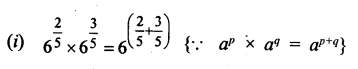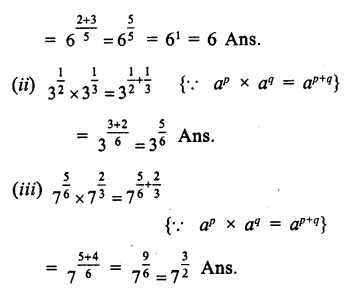Question 2.
Solution:
We know that
ap ÷ aq = ap-q
Therefore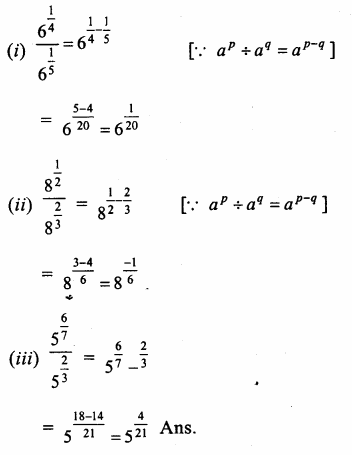Question 3.
Solution:
We know that
ap x bp = (ab)p
Therefore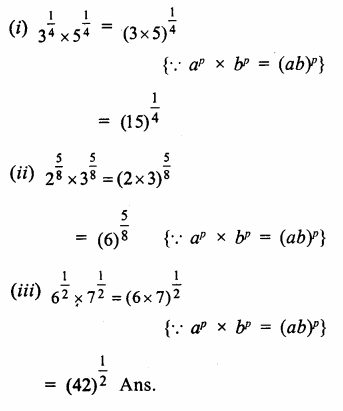Question 4.
Solution:
We know that
(ap)q =apq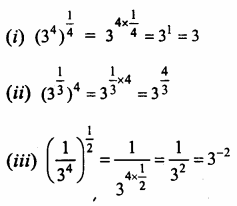Question 5.
Solution: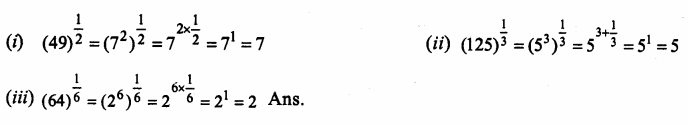Question 6.
Solution:Question 7.
Solution: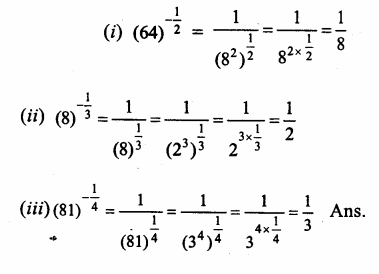Hope given RS Aggarwal Solutions Class 9 Chapter 1 Real Numbers Ex 1F are helpful to complete your math homework.

If you have any doubts, please comment below. Learn Insta try to provide online math tutoring for you.